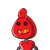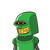# c) The present ages of Ram and Shyam are in the ratio of 5:3, respectively. Three yearslater, the ratio of their ages will b

c) The present ages of Ram and Shyam are in the ratio of 5:3, respectively. Three years
later, the ratio of their ages will become 11:9, respectively. What is Ram and Shyam’s
present age in years?

### 2 thoughts on “c) The present ages of Ram and Shyam are in the ratio of 5:3, respectively. Three years<br />later, the ratio of their ages will b”

1.The present age of Ram = 5x = 5×1/2 = 2.5 years. The present age of Shyam = 3x =3×1/2 = 1.5 years.

2.• The present age of Ram is 2.5 years. and
• The present age of Shyam is 1.5 years.

Step-by-step explanation:

### Given that:

• The present ages of Ram and Shyam are in the ratio of 5:3,
• Three yearslater, the ratio of their ages will become 11:9

### To find:

• Ram and Shyam’spresent age in years?

### Solution:

Let the present age of Ram be 5x

Let the present age of Shyam be x

three years later

Age of Ram became = 5x+3

Age of Shyam became = 3x+3

$$= > \frac{5x + 3}{3x + 3} = \frac{11}{9} \\ = > 45x + 27 = 33x + 33 \\ = > 45x – 33x = 33 – 27 \\ = > 12x = 6 \\ = > x = \frac{6}{12} \\ = >x = \frac{1}{2} \\$$

Now

The present age of Ram = 5x => 5×½ => 2.5 years.

The present age of Shyam => 3x => 3×½ => 1.5 years.

$\fbox{${\fbox{${{I}\hspace{0.33em}{hope}\hspace{0.33em}{its}\hspace{0.33em}{help}\hspace{0.33em}{you}}$}}$}$